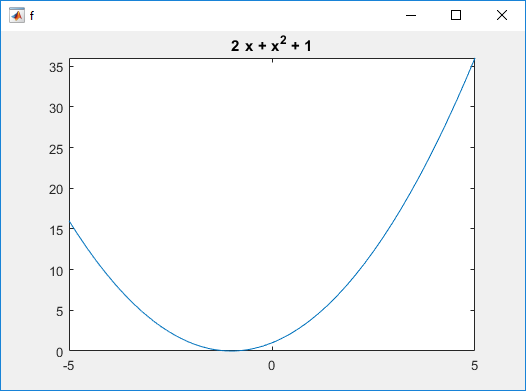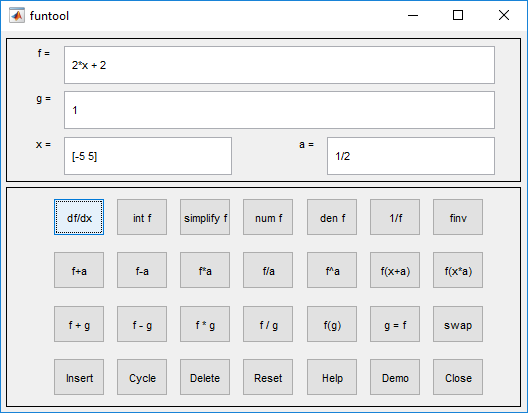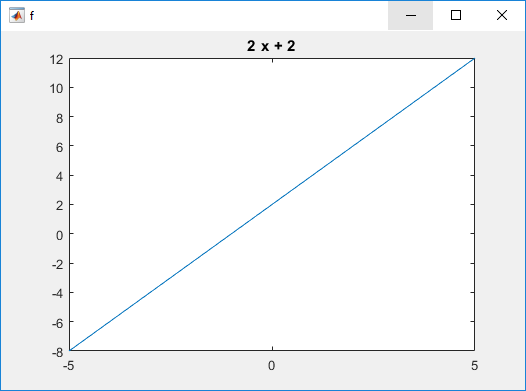# funtool

Function calculator

## Description

The `funtool` app is a visual function calculator that manipulates and displays functions of one variable. At the click of a button, for example, `funtool` draws a graph representing the sum, product, difference, or ratio of two functions that you specify. `funtool` includes a function memory that allows you to store functions for later retrieval.

## Open the funtool

At startup, `funtool` displays graphs of a pair of functions, `f(x) = x` and `g(x) = 1`. The graphs plot the functions over the domain `[-2*pi, 2*pi]`. `funtool` also displays a control panel that lets you save, retrieve, redefine, combine, and transform `f` and `g`.The top of the control panel contains a group of editable text fields.

 f= Displays a symbolic expression representing `f`. Edit this field to redefine `f`. g= Displays a symbolic expression representing `g`. Edit this field to redefine `g`. x= Displays the domain used to plot `f` and `g`. Edit this field to specify a different domain. a= Displays a constant factor used to modify `f` (see button descriptions in the next section). Edit this field to change the value of the constant factor.

`funtool` redraws `f` and `g` to reflect any changes you make to the contents of the control panel's text fields.

The bottom part of the control panel contains an array of buttons that transform `f` and perform other operations.

The first row of control buttons replaces `f` with various transformations of `f`.

df/dx

Derivative of `f`

int f

Integral of `f`

simplify f

Simplified form of `f`, if possible

num f

Numerator of `f`

den f

Denominator of `f`

1/f

Reciprocal of `f`

finv

Inverse of `f`

The operators int f and finv can fail if the corresponding symbolic expressions do not exist in closed form.

The second row of buttons translates and scales `f` and the domain of `f` by a constant factor. To specify the factor, enter its value in the field labeled a= on the calculator control panel. The operations are

 f+a Replaces `f(x)` by ```f(x) + a```. f-a Replaces `f(x)` by ```f(x) - a```. f*a Replaces `f(x)` by ```f(x) * a```. f/a Replaces `f(x)` by ```f(x) / a```. f^a Replaces `f(x)` by ```f(x) ^ a```. f(x+a) Replaces `f(x)` by ```f(x + a)```. f(x*a) Replaces `f(x)` by ```f(x * a)```.

The first four buttons of the third row replace `f` with a combination of `f` and `g`.

 f+g Replaces `f(x)` by ```f(x) + g(x)```. f-g Replaces `f(x)` by `f(x)-g(x)`. f*g Replaces `f(x)` by ```f(x) * g(x)```. f/g Replaces `f(x)` by ```f(x) / g(x)```.

The remaining buttons on the third row interchange `f` and `g`.

 g=f Replaces `g` with `f`. swap Replaces `f` with `g` and `g` with `f`.

The first three buttons in the fourth row allow you to store and retrieve functions from the calculator's function memory.

 Insert Adds `f` to the end of the list of stored functions. Cycle Replaces `f` with the next item on the function list. Delete Deletes `f` from the list of stored functions.

The other four buttons on the fourth row perform miscellaneous functions:

 Reset Resets the calculator to its initial state. Help Displays the online help for the calculator. Demo Runs a short demo of the calculator. Close Closes the calculator's windows.

## Examples

expand all

Display a quadratic function by editing the text field ```f = x^2 + 2*x + 1```. Plot the quadratic function within the interval ```x = [-5 5]```.Click df/dx to find the derivative of the quadratic function.